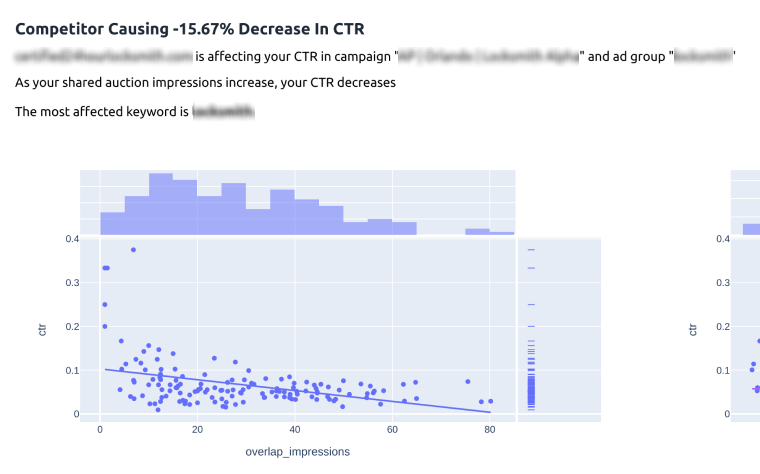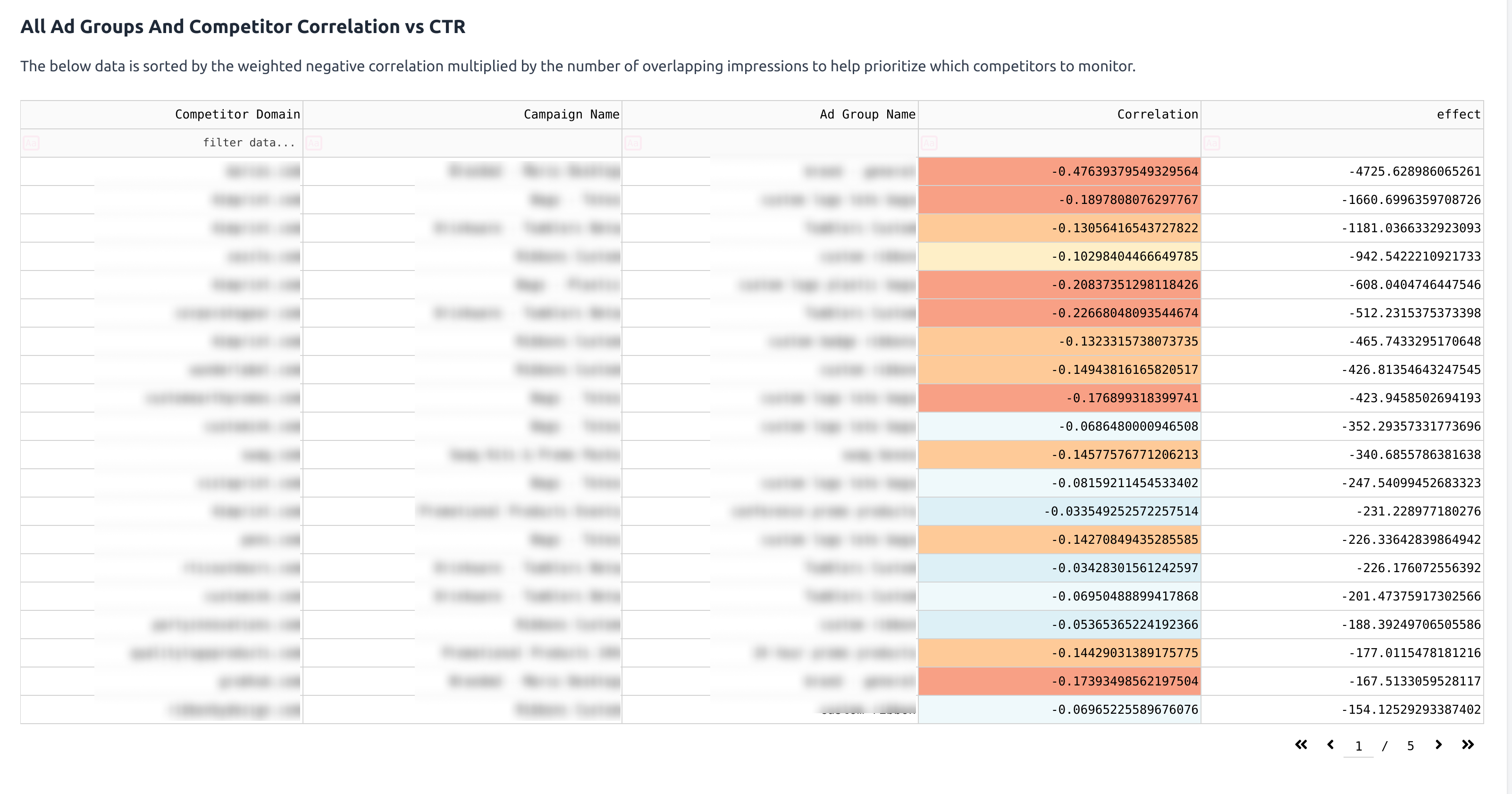As far as I am aware, there has never been a commercially available solution to generate a report of this kind.

The reason being, Google doesn’t allow you to access auction insights data via the API.

I’ve spoken to at least one data scientist at a big Google Ads agency that has run a report like this. So while this may be the first time an article has been published, I certainly was not the first to generate such insights.Here’s the summary:

Through exporting Google Ads auction insight data day-by-day, you can monitor the changing overlap rate of competitors compared to your CTR and CPA.

It makes sense, right? If someone writes a better ad than you, and they show up more frequently, they are going to be more likely to win the click.

Or at least, if the user clicks on both of you, the better experience/offer will win the sale.

Is it rigorously scientific? No. Is it the best you’ll get. Yes.

In this article I will talk briefly about why you would want this, and exactly how to do it.

For those who would prefer to watch about what this does, here’s a quick video where I built this report into a now-sunset product:

### Why do this?

If you are testing your Google Ads (which you should be), it would be helpful to know what you’re testing against. Understanding which competitors are most effective will influence your testing.

You may also find instances where you are advertising for “wrong fit” keywords. For example, this report may show you competing against Amazon when you are doing lead generation.

There may be a new “upstart” competitor that is the cause of decreased performance, rather than “market conditions.”

Or you might find nothing (I doubt it though)

### Show me the code!

As with anything I write about, I have published the code here.

#### Exporting the right data

Because this data isn’t accessible from the Google Ads API, we have to export it via the report editor.

There are two different reports you need to generate:

Video instructions here:

A performance report:

• Campaign
• Campaign ID
• Keyword
• Keyword ID
• Search keyword
• Day

And these columns

• Cost
• Conversions
• Impr.
• Clicks
• Conv. value (if useful to you)

An auction insight report:

• Campaign
• Campaign ID
• Keyword
• Keyword ID
• Search keyword
• Day

And these columns

• Display URL Domain
• Search Impr. share (Auction Insights)
• Search overlap rate
• Position above rate
• Top of page rate
• Abs. Top of page rate

### Let’s walk through the code!

The entrypoint of the function shows how the general steps taken to generate this report:

``````def run_comp_analysis(performance_data_csv: str, auction_data_csv: str):
# Load the CSV exports and clean the columns
performance_data_df = load_csv(performance_data_csv, ['Search overlap rate', 'Position above rate', 'Top of page rate', 'Search outranking share', 'Abs. Top of page rate', 'Search Impr. share (Auction Insights)'])
auction_data_df = load_csv(auction_data_csv, ['Search overlap rate', 'Position above rate', 'Top of page rate', 'Search outranking share', 'Abs. Top of page rate', 'Search Impr. share (Auction Insights)'])

# Merge the two reports
merged_data = merge_performance_auction_data(performance_data_df, auction_data_df)

# Run the correlation analysis
df=merged_data,
filter_keys=get_top_n(merged_data, 'Ad group ID', 10, 'Cost'),
metric='ctr',
)
df=merged_data,
filter_keys=get_top_n(merged_data, 'Ad group ID', 10, 'Cost'),
metric='cpa',
)``````

Let’s break each of these steps down.

Data scientists do two things: clean data, and complain about cleaning data.

This first step prepares our dataframes nice and cleanly to convert strings to floats and parse dates.

``````def clean_percents(row):
if '< 10' in row:
return .1
if row == " --":
return 0
return float(row.rstrip('%')) / 100

def load_csv(file_name: str, clean_percent_names: list[str]) -> pd.DataFrame:
converter = {key: clean_percents for (key) in clean_percent_names}

#### Merging the data

As you recall, you had to export two different reports. One performance report, and another an auction insights report.

This merge step brings those two reports together by day, so you we will have the performance metrics and the auction insights metrics on the same row.

This way we can see, on each given day, how related are the fluctuations in CTR / CPA to the overlap rate (and more).

 Day Ad group CTR Display URL Domain Search overlap rate 2023-07-05 Google Ads Agency .0243 competitor.com 0.12
``````def merge_performance_auction_data(performance: pd.DataFrame, auction: pd.DataFrame) -> pd.DataFrame:
merged_data = pd.merge(left=performance, right=auction, on=['Day', 'Ad group ID', 'Keyword ID'])
merged_data['ctr'] = merged_data['Clicks'] / merged_data['Impr.']
merged_data['cpa'] = merged_data['Cost'] / merged_data['Conversions']
merged_data['overlap_impressions'] = merged_data['Search overlap rate'] * merged_data['Impr.']
merged_data.set_index('Day')
merged_data.fillna(0)
return merged_data.reindex(sorted(merged_data.columns), axis=1)``````

#### Running the correlation analysis

Now to the fun part! I’ll break this into a few steps.

Let’s revisit the function call. We have 5 arguments:

``````ad_group_ctr = get_corr_data(
df=merged_data,
filter_keys=get_top_n(merged_data, 'Ad group ID', 10, 'Cost'),
metric='ctr',
)``````

1: The dataframe (aka merged data)

2: filter_keys

This function isn’t vectorized, meaning that unless we’re careful, it can run fairly slow. (If anyone has a way to speed it up, email me!).

The get_top_n function tells the correlation analysis to only run on the top 10 ad groups by spend. You’re able to configure this however you’d like.

3: key_name

This analysis can be run on a campaign, ad group or keyword level. I tend to run it on the ad group level to get enough data. To ensure there are no naming collisions (like two ad groups being named the same), I use the ad group Id.

4: col_names_to_keep

Because we’re running on the “Ad group ID” (which is just a bunch of numbers), I specify I want to keep the actual names of the campaign and ad group associated with it.

#### Running the correlation

Here’s the meat of the logic.

I’ll break down each step.

``````def run_correlation(df: pd.DataFrame, identifier_list: list[str], identifier_key: str, metric: str) -> pd.DataFrame:
df = df[[identifier_key, metric, 'Search overlap rate', 'Position above rate', 'Impr.', 'overlap_impressions',
'Display URL domain']]
df = df[df[identifier_key].isin(identifier_list)]
df = df[(df['overlap_impressions'] != 0)]

if metric == 'cpa':
df = df.replace([np.inf, -np.inf], np.nan)
df = df.dropna(how='all')

filtered_df = df.groupby(by=['Display URL domain', identifier_key]).filter(lambda x: len(x) > 10)
groups = filtered_df.groupby(by=['Display URL domain', identifier_key])
corr_df = groups.apply(lambda x: pd.Series({
'display_url_domain': x['Display URL domain'].iloc,
'id': x[identifier_key].iloc,
'search_overlap_rate_correlation_weighted': WeightedCorr(x=x['Search overlap rate'], y=x[metric],
w=x['Impr.'])(),
'overlap_impressions': x['overlap_impressions'].sum()
}))
# corr_df = pd.DataFrame(result_list)
corr_df = corr_df.reset_index(drop=True)
return corr_df.sort_values(by=['overlap_impressions'], ascending=False)``````

1: Clean our dataframe

Yep, still need to clean the dataframe! This brings our dataframe down to the lowest amount of columns we need.

``````# Remove excess columns
df = df[[identifier_key, metric, 'Search overlap rate', 'Position above rate', 'Impr.', 'overlap_impressions',
'Display URL domain']]
df = df[df[identifier_key].isin(identifier_list)]

# Drop empty rows
df = df[(df['overlap_impressions'] != 0)]

# Because CPA can be "infinity" due to divide by zero issues, we drop all of these rows
if metric == 'cpa':
df = df.replace([np.inf, -np.inf], np.nan)
df = df.dropna(how='all')``````

2: Group our data and execute

The fastest way I found to run this calculation is through a “groupby + apply” pattern. Again, this isn’t vectorized so it’s a bit slower, but 20 hours of trying to vectorize this function was tough enough!

The below code groups by the competitor URL domain and our identifierkey (ad group ID). And then it applies a lambda function to each group.

``````groups = filtered_df.groupby(by=['Display URL domain', identifier_key])
corr_df = groups.apply(lambda x: pd.Series({
'display_url_domain': x['Display URL domain'].iloc,
'id': x[identifier_key].iloc,
'search_overlap_rate_correlation_weighted': WeightedCorr(x=x['Search overlap rate'], y=x[metric],
w=x['Impr.'])(),
'overlap_impressions': x['overlap_impressions'].sum()
}))``````

Let’s go through the lambda function step by step:

1: Group the data by the competitor’s URL and our identifier key (in this example that was the ad group ID)

``groups = filtered_df.groupby(by=['Display URL domain', identifier_key])``

2: Apply a lambda function to each group

``corr_df = groups.apply(lambda x: pd.Series({``

3: Pass through the arguments

The Display URL domain of the row

``'display_url_domain': x['Display URL domain'].iloc,``

The identifier (ad group ID in our case)

``'id': x[identifier_key].iloc,``

4: Run the correlation calculation

I use this lovely library to run the correlation analysis.

It’s important that we’re including the weight by impressions. Days where there were many more impressions from a competitor are much more likely to influence or metrics.

``````'search_overlap_rate_correlation_weighted': WeightedCorr(x=x['Search overlap rate'], y=x[metric],
w=x['Impr.'])(),``````

5: Calculate the overlap impressions

This is used as a sort key later on.

``'overlap_impressions': x['overlap_impressions'].sum()``

Return our dataframe

Here, we reset our index and return the dataframe by the competitors who had the most overlapping impressions on a given merge key.

``````corr_df = corr_df.reset_index(drop=True)
return corr_df.sort_values(by=['overlap_impressions'], ascending=False)``````

#### Final cleaning steps

At this point in our function, we have all the data we need.

However, all the data is tied to the “Merge Key” which in our case was an ad group ID.

I won’t include the function in this post, but you can see where I add back the names of the campaign and ad groups here.

Lastly, we sort the dataframe by the “north star metric” of impact.

We multiply the correlation by the number of overlap impressions to find the competitors that had the most impact.

For example, if a competitor has a negative 10% impact on your CTR and shows up with you 90% of the time, that competitor will be shown above another who might have a 50% impact on your CTR but shows up rarely.

``````def sort_by_impact(df: pd.DataFrame, impact_col_name: str, weight_col_name: str, nrows: int,
higher_is_better: bool) -> pd.DataFrame:
df['effect'] = df[impact_col_name] * df[weight_col_name]
if higher_is_better:
return df.sort_values(by=['effect'])[:nrows]
else:
return df.sort_values(by=['effect'], ascending=False)[:nrows]
``````

### The final result

Here’s a sample of a report generated.

You can see that the first row, there’s a competitor that causes a 47% decrease in CTR. This is an unfortunate brand name collision.

The next row shows another competitor selling a similar product at a lower price, resulting in an 18.9% decrease in CTR.

What will you find?